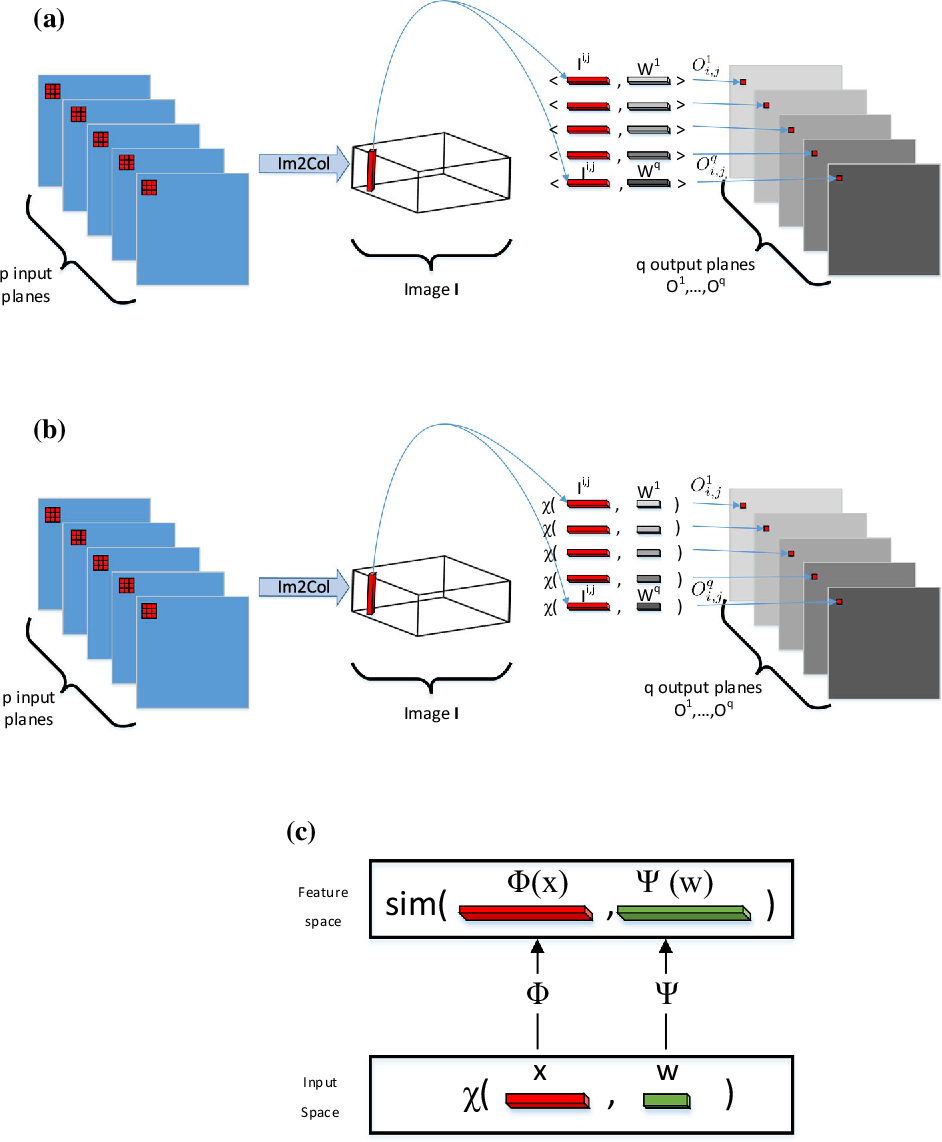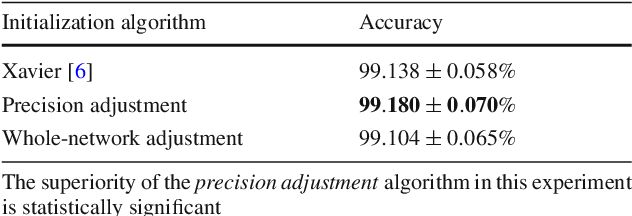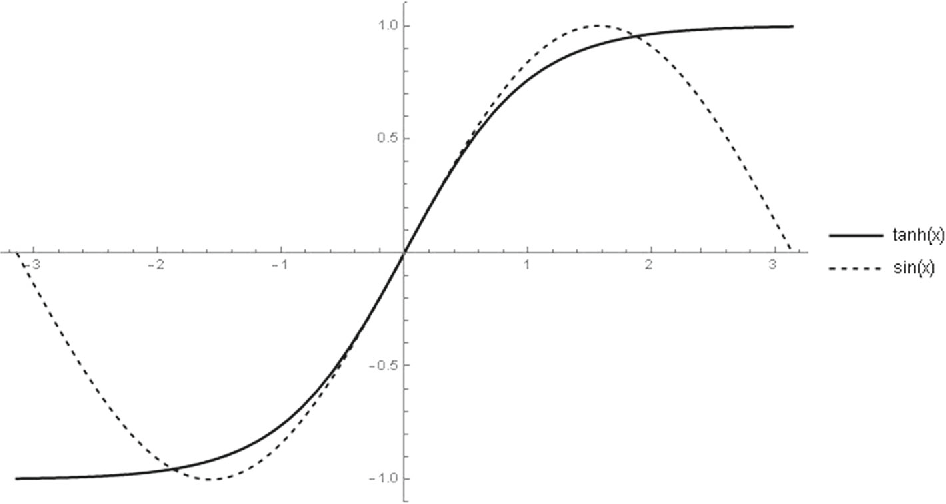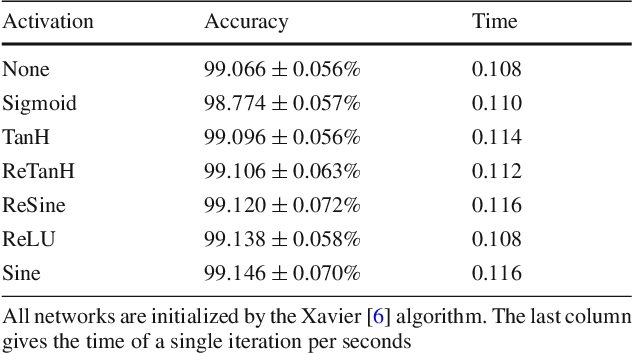Convolutional neural networks have become a main tool for solving many machine vision and machine learning problems. A major element of these networks is the convolution operator which essentially computes the inner product between a weight vector and the vectorized image patches extracted by sliding a window in the image planes of the previous layer. In this paper, we propose two classes of surrogate functions for the inner product operation inherent in the convolution operator and so attain two generalizations of the convolution operator. The first one is the class of positive definite kernel functions where their application is justified by the kernel trick. The second one is the class of similarity measures defined based on a distance function. We justify this by tracing back to the basic idea behind the neocognitron which is the ancestor of CNNs. Both methods are then further generalized by allowing a monotonically increasing function to be applied subsequently. Like any trainable parameter in a neural network, the template pattern and the parameters of the kernel/distance function are trained with the back-propagation algorithm. As an aside, we use the proposed framework to justify the use of sine activation function in CNNs. Our experiments on the MNIST dataset show that the performance of ordinary CNNs can be achieved by generalized CNNs based on weighted L1/L2 distances, proving the applicability of the proposed generalization of the convolutional neural networks.Click To Get Code For Paper